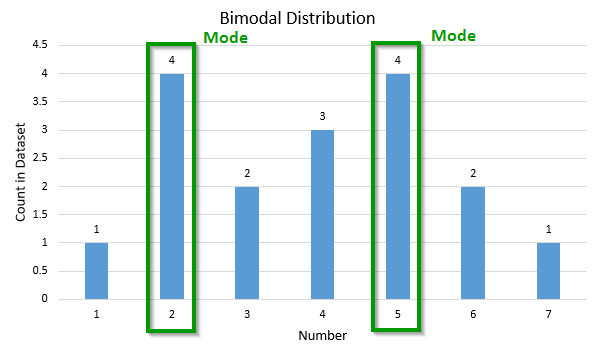# Mode Calculator

Use this mode calculator to easily calculate the mode of a set of numbers. Easily find the mode of an unimodal distribution or the modes of bimodal and multimodal sets.

Share calculator:

Embed this tool:
get code

## What is a mode?

The mode is a descriptive statistic, used as a summary measure for a set of data, and it represents the most often occurring element of the set. For example, in the set of numbers 1, 2, 3, 3, 4, 6, 10 the mode is 3, as it occurs twice and all the other numbers occur just once. A set of numbers can be bimodal or multimodal - have two or more modes, for example the set 1, 2, 3, 3, 4, 6, 10, 10 will have modes 3 and 10 (confirmed by our mode calculator).

The mode is the most frequent value in a set and is one of the three most used statistics, the other ones being the arithmetic mean and median. From the three, the mode is usually the least affected by outliers and extreme values. As a descriptive statistic it is minimizing the number of times a number from a set is not equal to that set's mode.

## How to find the mode?

To find the mode of a set of numbers, follow these three steps:

1. Order the numbers by value. This will result in all number of the same value being next to each other.
2. Count how many numbers are there for each distinct value.
3. Sort the counts from highest to lowest. The highest count is the mode.

### Can there be more than one mode?

A complication arises if there are two or more equal counts in which case there can be two or more modes. A set with two modes is called bimodal, a set with more than two modes is called multimodal. Here is an example of a bimodal distribution of numbers:This example set of numbers contains the following numbers (sorted): 1, 2, 2, 2, 2, 3, 3, 4, 4, 4, 5, 5, 5, 5, 6, 6, 7. A mode finder would highlight two modes at the numbers two and five.

## Practical applications and examples

Mode calculation makes sense for non-numerical (e.g. categorical) data, like names or family names, professions, car models, etc. Most voting systems in the world have at their core the mode of the set of votes - counting who got the most votes, whose name appears most frequently in the set of votes cast, these are all different ways to describe the mode. See When to Use Mean, Median, or Mode for a longer discussion on when to use the mode of a set.

An example from the application of probability theory: if you know that the mode of a set is "5", and you have to bet on what the value of a randomly drawn element from the set will be, the mode is your best bet. Using an online calculator you can easily calculate the arithmetic mode for even a large dataset.

#### Cite this calculator & page

If you'd like to cite this online calculator resource and information as provided on the page, you can use the following citation:
Georgiev G.Z., "Mode Calculator", [online] Available at: https://www.gigacalculator.com/calculators/mode-calculator.php URL [Accessed Date: 01 Apr, 2023].